# RFEM – Online Manual

Online manuals, introductory examples, tutorials, and other documentation.

# 7.3.3 Global Calculation Parameters

### Global Calculation Parameters

The Global Calculation Parameters dialog tab manages the settings that are generally applied to all load cases and load combinations. To open the corresponding dialog box, select

• Calculate → Calculation Parameters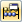in the menu or use the corresponding toolbar button.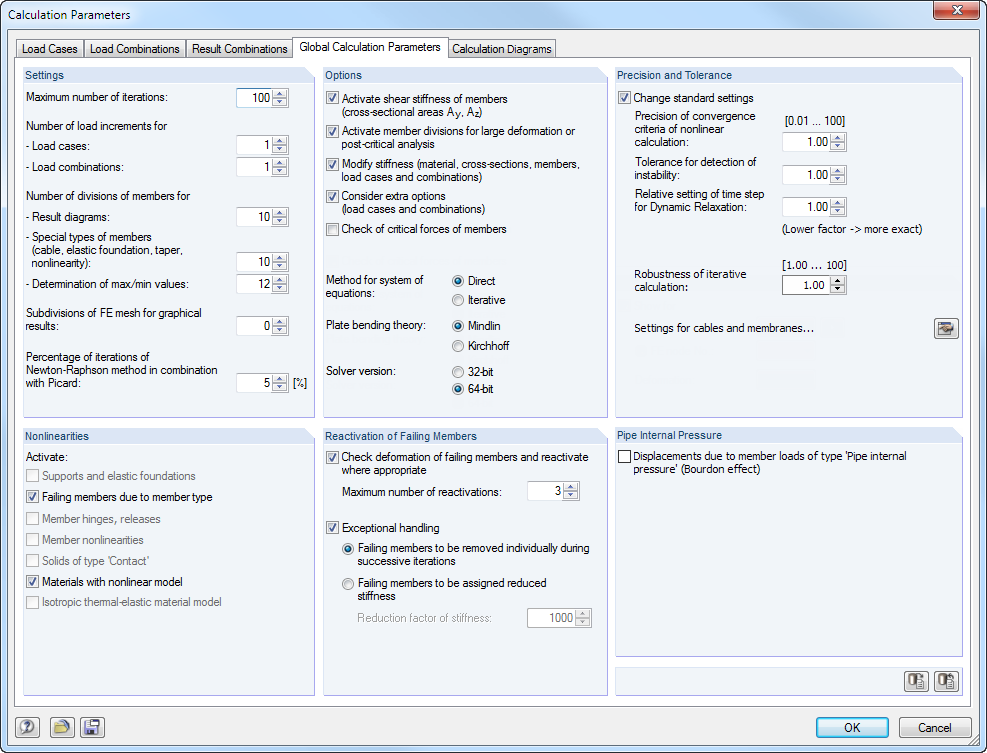Figure 7.25 Calculation Parameters dialog box, Global Calculation Parameters tab
Settings
Maximum number of iterations

When using the second-order or large deformation analysis as well as objects that are nonlinearly effective, you have to calculate iteratively. The value of the text box defines the highest possible number of calculation runs. The specification has nothing to do with the iterative method set for the system of equations described in the Options dialog section.

When the calculation reaches the maximum number of iterations without achieving an equilibrium, RFEM displays a corresponding message. The results can then be displayed regardless.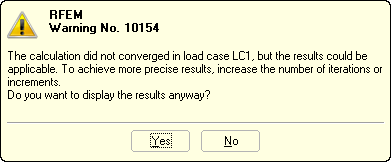Figure 7.26 Message displayed for convergence problem
Number of load increments

Specifications of this text box only take effect for calculations according to the second-order or large deformation analysis. Finding an equilibrium is often difficult when large deformations are considered. Instabilities can be avoided by applying the load in several steps.

For example, if two load increments are specified, half of the load is applied in the first step. Iterations are carried out until the equilibrium is found. Then, in the second step, the complete load is applied to the already deformed system and iterations are run again until the state of equilibrium is reached.

Please keep in mind that load increments have an unfavorable effect on the computing time. A value of 1 (no gradual load increment) is therefore preset in the text box.

Moreover, you can define how many load increments you want to apply for each load case and combination separately (see Chapter 7.3.1.1). The global specifications are then ignored.

Number of divisions of members for result diagrams

This text box affects the graphical result diagram of members that have no other, distinct FE mesh division (due to an FE mesh refinement or a connected surface, for example). If a division of 10 is set, RFEM divides the length of the longest member in the system by 10. With this system-related division length, RFEM determines the graphical result distributions on the division points for each member.

In the FE Mesh Settings dialog box, there is another division option for straight members that are not integrated in surfaces (see Figure 7.10). With this option, you can create FE nodes on all free members whose results are used for the graphical result diagrams.

Number of divisions of members for special types of members (cable, etc.)

In contrast to the previous division option, a real division of the member is defined by internal intermediate nodes. This specification affects cables, foundation members (contact stresses), tapered members (interpolation of cross-section values), and members with plastic properties (yielding zones), if they have not yet been divided otherwise by FE nodes: This division is irrelevant if a member is placed on a boundary line of a surface or if the definition line has an FE mesh refinement.

Number of divisions of members for determination of max/min values

This value specifies the internal division that the maximum and minimum internal forces of members are determined with. Thus, the division (default setting: 12) represents the basis for the extreme values shown in the results tables and graphic. The division is also used for calculating member internal forces of load combinations.

Subdivisions of FE mesh for graphical results

This controls the accuracy of graphical distributions within the finite elements. The following example compares the results with the divisions 0 and 3.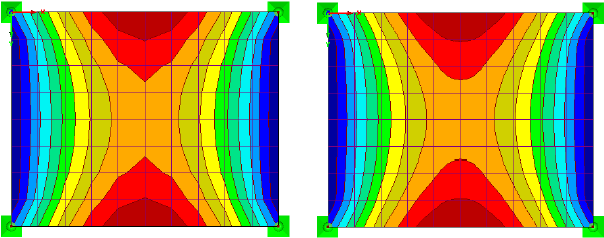Figure 7.27 Graphical result diagram m-x with division 0 (left) and 3 (right)
Percentage of iterations of Newton-Raphson method in combination with Picard

The approach according to Picard acts on the assumption of secant stiffenings, while the method of Newton-Raphson assumes tangent stiffenings (see Chapter 7.3.1). When the calculation option Newton-Raphson combined with Picard is selected, secant stiffenings are used in the first iterations before tangent stiffenings are applied for the remaining iterations.

In the text box, you can define the percentage of the first iterations with secant stiffenings. Specify the value in relation to the total number of iterations.

Options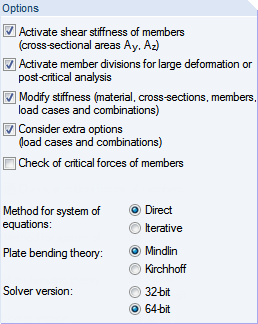Activate shear stiffness of members (cross-sectional areas Ay, Az)

Considering shear stiffnesses leads to an increase of deformations due to shear forces. The shear deformation is hardly relevant for rolled and welded cross-sections. For solid and timber cross-sections, however, it is recommended to consider the shear stiffnesses for the deformation analysis.

Activate member divisions for large deformation or post-critical analysis

Beams can be divided by intermediate nodes for the calculation according to the large deformation analysis to calculate such members with a higher accuracy. The number of divisions is taken from the text box for cable and foundation members.

Modify stiffness (material, cross-sections, members, load cases and combinations)

Use this check box to globally define whether the adjustment factors of the stiffnesses for materials (see Chapter 4.3), cross-sections (see Chapter 4.13), and members (see Chapter 4.17) are considered in the calculation of load cases and load combinations. In the member and cross-section dialog boxes, factors of 1.00 are respectively preset. Thus, the check mark in the check box usually does not result in reductions or increases of the stiffnesses.

Consider extra options

If Extra Options have been defined for the calculation parameters of load cases and combinations (see Chapter 7.3.1.3), it is possible to globally activate or deactivate them with this check box.

Check of critical forces of members

Exceeding the critical load often leads to an instability message as early as in the first iteration. Use this check box to control if the critical load is checked for trusses, compression members, and buckling members. The defined effective lengths of members will be taken into account.

Method for system of equations

Both options control the method used for solving the equation system: Direct or Iterative. To prevent misunderstandings: When solving the equation system directly, an iterative calculation is performed as well, if there are nonlinearities or if data is calculated according to the second-order or large deformation analysis. Direct and Iterative refer to the data management during the calculation.

Which solver method leads to results more quickly depends on the complexity of the model as well as the size of the available main memory (RAM):

• For small and medium-sized systems, the Direct solver method is more effective.
• For large and complex systems, the Iterative method leads to results more quickly.

Once the matrices for the direct method can no longer be stored in the main memory, Windows starts to swap parts of the data to the hard disk, which slows down the calculation. Hard disk activities increase and the processor load is reduced, which is visible in the Windows Task Manager. By using the iterative ICG (Incomplete Conjugate Gradient) calculation method, you can avoid this storage problem.

It is necessary to ensure that the swap file is large enough or the file size is assigned automatically by Windows. When a swap file is too small, program crashes may occur.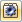Use the menu item Options → Program Options or the toolbar button shown on the left to open the Program Options dialog box. In the Help Assistant dialog tab, you can define the number of 2D and 3D elements that must be exceeded for RFEM to show a warning before the calculation with the direct method.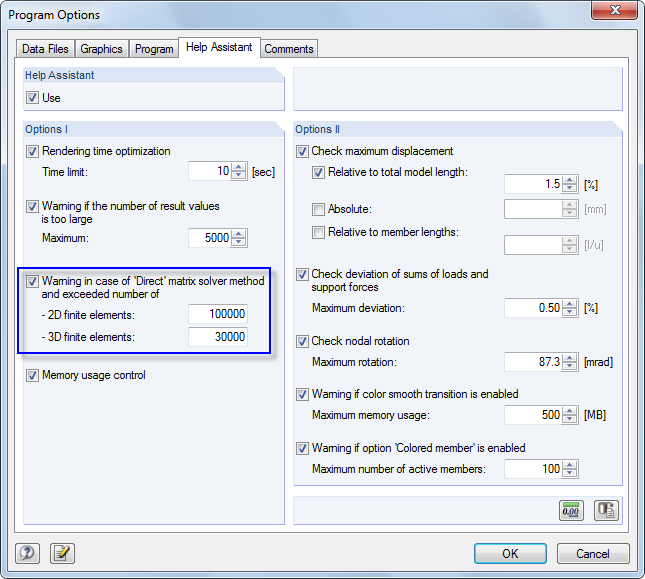Figure 7.28 Program Options dialog box, Help Assistant tab
Plate bending theory

Surfaces can be calculated according to the bending theories of Mindlin or Kirchhoff. The calculation according to Mindlin includes shear force deformations; in the calculation according to Kirchhoff, they are not considered. The Mindlin calculation option is therefore suitable for relatively thick plates and shells used in solid construction, while the Kirchhoff option is recommended for surfaces that are relatively thin such as metal sheets in steel construction.

Solver version

The direct method for solving the system of equations (see above) is based on an analysis core using the extended RAM memory capacities of 64-bit operating systems. This makes it possible to efficiently calculate all load cases and load combinations at once even for big structural systems, provided that no object nonlinearities become effective. The RAM memory must be large enough to cover the stiffness matrix and all loading.

Precision and Tolerance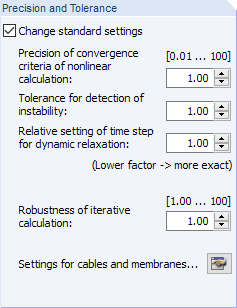Only rarely is it necessary to adjust the preset convergence and tolerance parameters. Select the Change standard settings check box to enable the text boxes below.

Precision of convergence criteria of nonlinear calculation

If nonlinear effects are involved or if the calculation is carried out according to the second-order or large deformation analysis, you can influence the calculation by means of convergence criteria.

The change of axial forces in the two last iterations is compared member by member. As soon as this change reaches a specific fractional amount of the maximum axial force, the calculation stops. During the iteration process, however, it is possible for axial forces to oscillate between two values instead of converging. A sensitivity can be defined with the value entered in this text box in order to neglect this oscillation effect.

The accuracy also affects the convergence criterion for deformation changes in calculations according to the large deformation analysis, where geometric nonlinearities are considered.

The default value is 1.0. The minimum factor is 0.01, the maximum value 100.0. The higher the factor, the less sensitive the break-off limit.

Tolerance for detection of instability

There are different approaches according to which the stability behavior of a model can be analyzed. However, none of them is able to detect singular stiffness matrices with absolute reliability.

RFEM uses two procedures to determine the instability: On the one hand, elements on the principal diagonal of the stiffness matrix are always compared with the same number in the iterations; on the other hand, each element of the principal diagonal is analyzed in relation to the adjacent number. The tolerance can be adjusted in the text box. The smaller the value is, the less sensitively the analysis is performed.

Relative setting of time step for Dynamic Relaxation

The time parameter controls the calculation as per the method of dynamic relaxation (see Chapter 7.3.1.1). The smaller the value, the smaller the time step and the more accurate the results.

Robustness of iterative calculation

In case of convergence problems with the Newton-Raphson method, you can increase the robustness to prevent the solution from being "skipped". It may be necessary to increase the maximum number of iterations.

Settings for cables and membranes

With the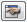button, a dialog box opens where you can activate an initial prestress for cables and membranes. Moreover, it is possible to consider the effect of membranes for compressive forces. In doing so, you can often improve the robustness of the calculation and the convergence behavior.

Nonlinearities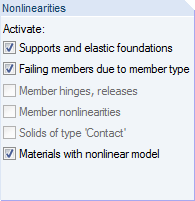If nonlinearly acting elements are used in the model, you can deactivate the effect of the following elements for the calculation:

The nonlinear effects should only be suppressed for testing purposes in order to find the cause of an instability, for example. The options in this dialog section help you to find errors: Incorrectly defined failure criteria are sometimes responsible for calculation break-offs.

Reactivation of Failing Members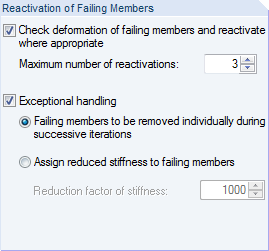Settings in this dialog section concern member elements that may fail (e.g. tension members, compression members, or foundation members). These options allow you to solve instability problems caused by failing members: A model is stiffened by ties, for example. Because of post shortenings due to vertical loads, the ties receive small compressive forces in the first calculation run. They are removed from the system. In the second calculation run, the model is unstable without these ties.

Check deformation of failing members and reactivate where appropriate

If this check box is selected, RFEM analyzes the nodal displacements in each iteration. If the member ends of a failed tie move away from each other, the member is reactivated.

In some cases, reactivating members may be problematic: A member is removed after the first iteration, reactivated after the second one, removed again after the third iteration, etc. The calculation would run this loop until it reaches the maximum number of iterations, without converging. This effect can be avoided by defining a Maximum number of reactivations, which specifies how often a member element is permitted to be reactivated before it is definitively removed from the stiffness matrix.

Exceptional handling

After selecting the check box, two methods for the handling of failing members are available for selection. They can be combined with the reactivation option described above.

• Failing members to be removed individually during successive iterations
• After the first iteration, RFEM does not, for example, remove all tension members with a compression force at once, but only the tie with the greatest compressive force. In the second iteration, only one member is then missing in the stiffness matrix. Subsequently, the tie with the greatest compressive force is removed again. Because of redistributing effects, a better convergence behavior can usually be achieved for the system in this way.
• This calculation option requires more time because the program must go through a larger number of iterations. Furthermore, you have to make sure that a sufficient number of possible iterations is set in the Settings dialog section above.
• Assign reduced stiffness to failing members
• The failed members are not removed from the stiffness matrix, but are instead assigned a very small stiffness. Specify it in the Reduction factor of stiffness text box: A factor of 1000 signifies a reduction of the stiffness to 1/1000.

For this calculation option, please keep in mind that RFEM displays small internal forces on members, which can technically not be absorbed by the member due to its definition.

Pipe Internal Pressure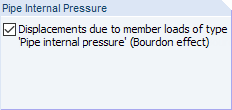The check box is important for the Pipe internal pressure member load. The so-called Bourdon effect describes the effort of a bent pipe to straighten under the influence of pressure. The perimeter stresses and axial stresses from internal pressure load lead to a longitudinal strain of the pipe when considering the material stiffness and transversal strain.

An example for calculating the pipe internal pressure is described in the following technical article:
https://www.dlubal.com/en/support-and-learning/support/knowledge-base/001102